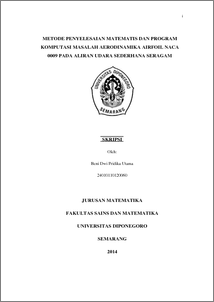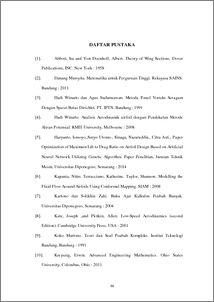# METODE PENYELESAIAN MATEMATIS DAN PROGRAM KOMPUTASI MASALAH AERODINAMIKA AIRFOIL NACA 0009 PADA ALIRAN UDARA SEDERHANA SERAGAM

Utama, Beni Dwi Pridika (2015) METODE PENYELESAIAN MATEMATIS DAN PROGRAM KOMPUTASI MASALAH AERODINAMIKA AIRFOIL NACA 0009 PADA ALIRAN UDARA SEDERHANA SERAGAM. Undergraduate thesis, UNDIP.Preview
PDF
933KbPDF
Restricted to Repository staff only

1872KbPreview
PDF
180Kb

## Abstract

Study of fluid flow problems in around the airfoil first use physical model of airfoil, then be examined in a wind tunnel. While to solve the control equation of fluid flow (i.e. Navier-Stokes equation) must be using the highest ability computer. This fulfillment so expensive. However, Navier-Stokes equation has simplified to Laplace equation as the control equation of incompressible, irrotational and inviscid flow. A partial differential equation with eliptic properties can be solved semi-analytically. Computational and mathematical method for completion Laplace equation and can be providing analysis of aerodinamica has been developed. First step in this method is fluid flow propagation analysis to obtaining colocations point on around of airfoil. So that the tangential component will be obtained. Next, from tangential component can be calculating potential velocity of uniform flow on each tangential component by integrating potential complex of uniform flow with vector position. Then, calculating of aerodynamic can be obtain by computational programming matlab. The results of this method should be compared to be validating the results of physic experiment from ref . Keywords : airfoil, Laplace equation, propagation vector, uniform flow fluid

Item Type: Thesis (Undergraduate) Q Science > QA Mathematics Faculty of Science and Mathematics > Department of Mathematics 84283 INVALID USER 14 Jun 2022 09:23 14 Jun 2022 09:23

Repository Staff Only: item control page